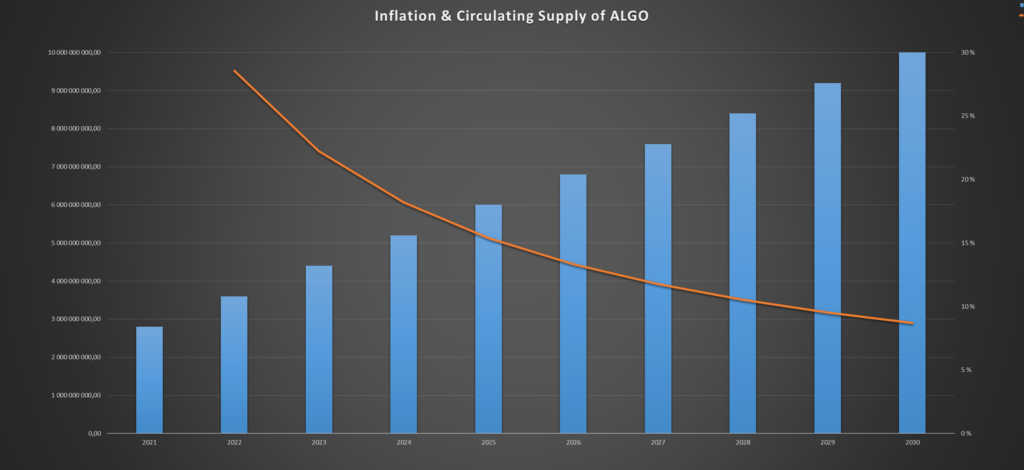Why is Algorand so cheap?

With its amazing technology, Algorand is becoming one of the most promising projects in the blockchain space, but why is Algorand so cheap?

Algorand is cheap because of the amount of circulating coins. The price is a function of market capitalization divided by supply. As ALGO has a huge supply, the price logically has to be low, as the value of ALGO is spread across billions of coins.

Let’s get into what this means, and why Algorand is so cheap compared to other coins.

The Reason Algorand is Priced so Low

As mentioned above, the price is a function of market cap divided by supply, but what exactly does that mean?

I’m going to show you step by step what I mean:

Let’s say, for example, there are only 100 ALGO coins in existence.

Also, that the “market capitalization”, the total value of all the coins combined, is \$200.

To calculate the price per coins, we now need to divide the market cap (total value) with the supply (number of coins):

\$200 / 100 ALGO = \$2/ALGO

With a supply of 100 ALGO, and a market cap of \$200, the price per ALGO has to be \$2.

In other words, the price is merely a representation of the relationship between the market cap and the supply of a specific coin.

The Huge Supply of Algorand Makes it Cheap

Now that you understand how coins are priced, and that supply is a big part of the equation, we can get into why Algorand is so cheap.

There are two kinds of supply; circluting supply and max supply. The circulating supply is the number of coins in circulation. The max supply is the “end game” supply. It is the number of coins that eventuellt will circulate.

The circulating supply of ALGO is currently 2.8 Billion coins. However, the max supply is 10 Billion. (Source: coinmarketcap and CoinGecko)

This means that over 7 Billion extra ALGO coins will come into circulation in the future.

“So what?”

As you know the price is calculated by dividing the market cap with the circulating supply. If the circulating supply increases, the price decreases.

From the image above, you can see that 74% of the ALGO supply is not in circulation yet. Another way to say this, is that the max supply is 3.57 times larger than the circulating supply.

If all this supply was to come into circulation tomorrow, how do you think this would affect the price of ALGO?

The price per token would decrease with roughly 74%.

To show this, we can run the same calculations as we did in the example from earlier, but with a 3.75x increase in supply:

\$200 / 357 ALGO = \$0.53/ALGO (\$0.53 is 26% of \$2)

The speed at which these coins are released is also interesting. This is known as the inflation rate of the token.

Will Algorand Continue Being Cheap in the Future? The Inflation of ALGO

According to the Algorand foundation, the initial plan was to distribute all the tokens within 2024.

This would have a dramatic effect on the price, as we saw above.

Therefore, they decided to lengthen it to 2030:

We have extended the distribution of the fixed and immutable total supply of 10bn Algo from 4 to 10 years from now, ending in 2030, rather than in 2024 as initially planned.

Algorand Foundation (2020)

In other words, within this decade Algorand will distribute roughly 7 200 000 000 ALGO, 3.57x’ing the circulating supply.

If we assume a constant rate of distribution, the average yearly increase in supply would be 7 200 000 000 ALGO / 9 years = 800 000 000 ALGO/year.

The inflation rate would decrease, as the circulating supply will grow each year, but the number of newly distributed coins remain constant:

As you can see, the inflation rate starts out at above 30%, but decreases exponentially, going below 10% in 2030.

This is relatively high, and will affect the price of ALGO drastically.

The takeaway from this:

The inflation rate of ALGO is 30%, and decreases exponentially down to 10% in 2030. If the market cap does not increase with 30% this year, and continues to grow at the same percentage as the inflation rate, the price per ALGO by necessity has to decrease.

How the Bitcoin Halving Affects the Price of Bitcoin

It goes into the inflation rate of Bitcoin, and how it’s the cornerstone of Bitcoin value.